## 14.6.3 Path-Constrained Trajectory Planning

This section assumes that a path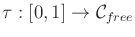has been given. It may be computed by a motion planning algorithm from Part II or given by hand. The remaining task is to determine the speed along the path in a way that satisfies differential constraints on the phase space. Assume that each state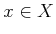represents both a configuration and its time derivative, to obtain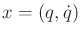. Letdenote the dimension of; hence, the dimension ofis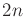. Once a path is given, there are only two remaining degrees of freedom in: 1) the position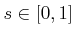along the domain of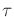, and 2) the speed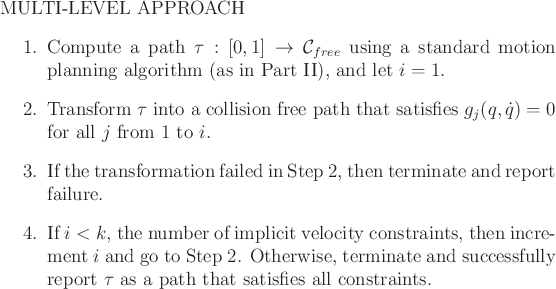at each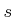. The full state,, can be recovered from these two parameters. As the state changes, it must satisfy a given system,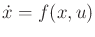. It will be seen that a 2D planning problem arises, which can be solved efficiently using many alternative techniques. Similar concepts appeared for decoupled versions of time-varying motion planning in Section 7.1. The presentation in the current section is inspired by work in time-scaling paths for robot manipulators [456,876,879], which was developed a couple of decades ago. At that time, computers were much slower, which motivated the development of strongly decoupled approaches.

Subsections
Steven M LaValle 2012-04-20